# Introduction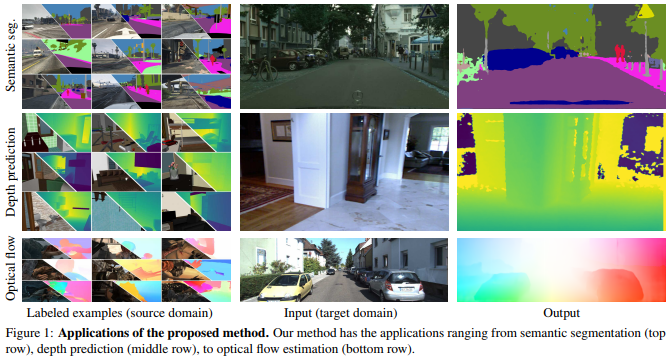This paper presents a novel pixel-wise adversarial domain adaptation algorithm by leveraging image-to-image translation methods for data augmentation. Because while the translated images between domains may differ in styles, their predictions for the task should be consistent. This method wants to exploit this property and introduce a cross-domain consistency loss that enforces the adapted model to produce consistent predictions.

CrDoCo is a pixel-level adversarial domain adaptation algorithm for dense prediction tasks. The model consists of two main modules:

• An image-to-image translation network.
• Two domain-specific task networks (one for source and the other for target).

The image translation network learns to translate images from one domain to another such that the translated images have a similar distribution to those in the translated domain. The domain-specific task network takes images of source/target domain as inputs to perform dense prediction tasks.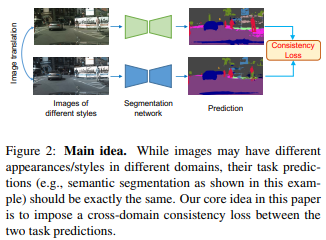As illustrated in the figure above, the idea is that while the original and the translated images in two different domains may have different styles, their predictions from the respective domain-specific task network should be exactly the same. This constraint is enforced using a cross-domain consistency loss that provides additional supervisory signals for facilitating the network training, allowing the model to produce consistent predictions.

# Method

In this setting, the source image is $$X_S$$, the source label $$Y_S$$, and an unlabeled target image $$X_T$$. The goal is to learn a task network $$F_T$$ that can reliably and accurately predict the dense label for each image in the target domain. They present an end-to-end trainable network which is composed of two main modules:

• The image translation network $$G_S \rightarrow T$$ and $$G_T \rightarrow S$$.
• Two domain-specific task networks $$F_S$$ and $$F_T$$.

The image translation network translates images from one domain to the other. The domain-specific task network takes input images to perform the task of interest.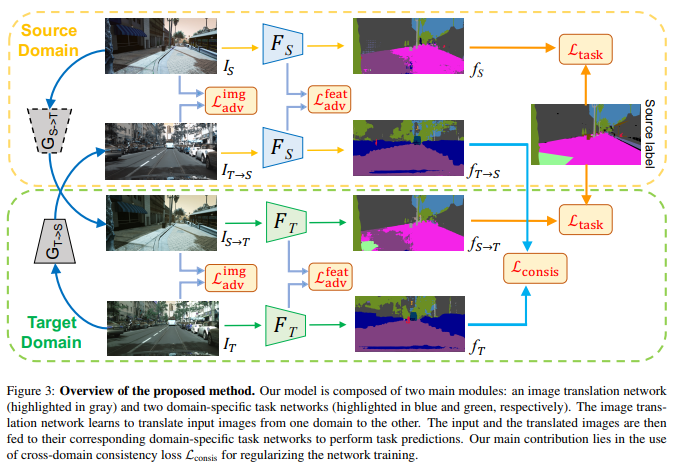The proposed network above takes an image $$I_S$$ from the source domain and another image $$I_T$$ from the target domain as inputs. They use the image translation network to obtain the corresponding translated images $$I_S \rightarrow T = G_S \rightarrow T (I_S)$$ (in the target domain) and $$I_T \rightarrow S = G_T \rightarrow S(I_T)$$ (in the source domain). Then pass $$I_S$$ and $$I_T \rightarrow S$$ to $$F_S$$, $$I_T$$ and $$I_S \rightarrow T$$ to $$F_T$$ to obtain their task predictions.

## Cross-domain consistency Loss

For semantic segmentation tasks, they use the bi-directional KL divergence loss and define the cross-domain consistency loss for semantic segmentation.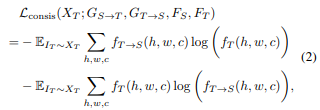This one is simply a cross-entropy loss between the task predictions and the corresponding ground truth labels as the task loss.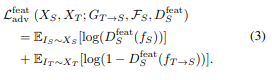This loss considers image-level adaptation between the translated images and those in the corresponding domain.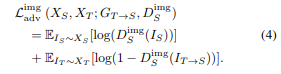## Reconstruction Loss

Here, they exploit the property that when translating an image from one domain to another, followed by performing a reverse translation, we should obtain the same image.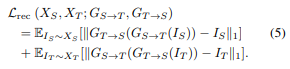# Results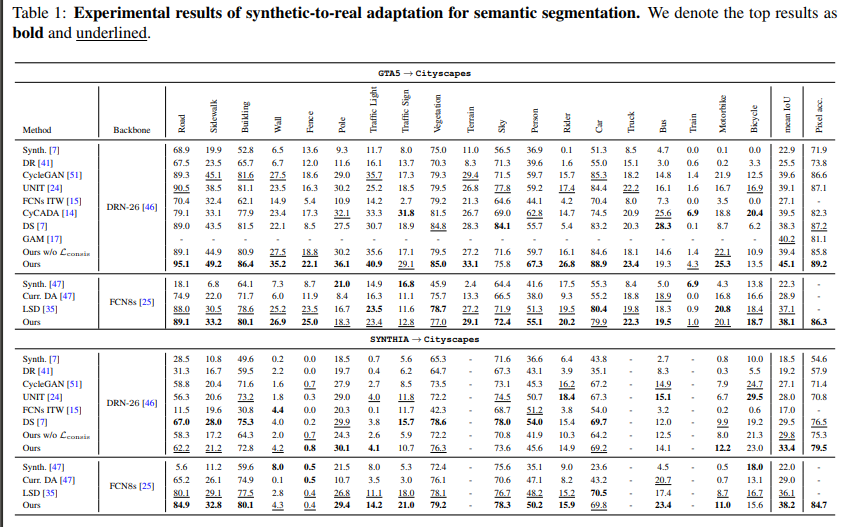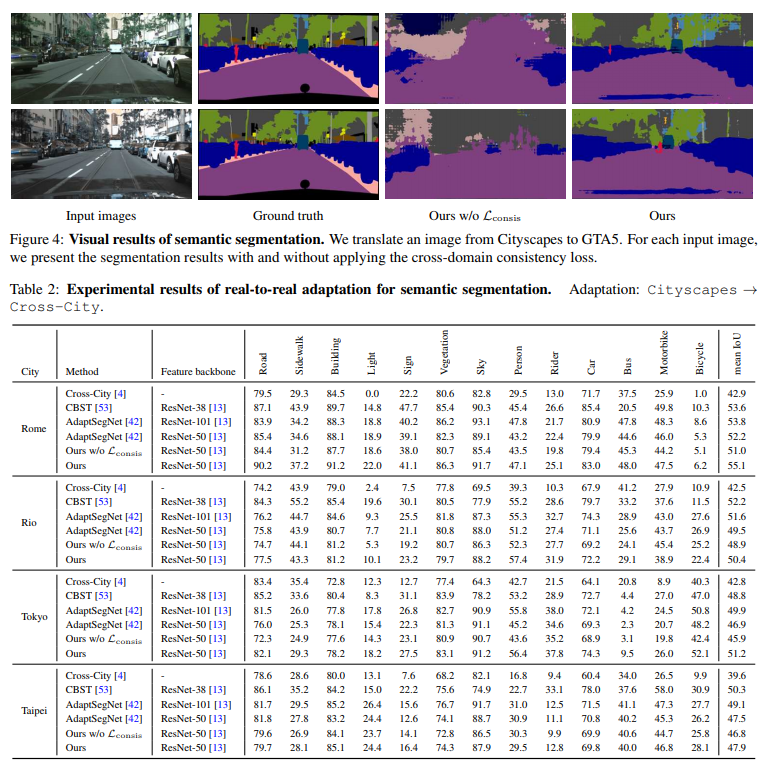# Limitations

This method is memory-intensive as the training involves multiple networks at the same time. Their suggested approches to this issue include adopting partial sharing on the two task networks, share the last few layers of the two task networks, and sharing the encoders in the image translation network.

# Conclusion

This paper presented an effective loss for improving pixel-level unsupervised domain adaption for dense prediction tasks. They show that by incorporating the proposed cross-domain consistency loss, the method consistently improves the performances over a wide range of tasks.